# Random Date Generator in Excel & Google Sheets

This tutorial will demonstrate how to generate random dates in Excel and Google Sheets.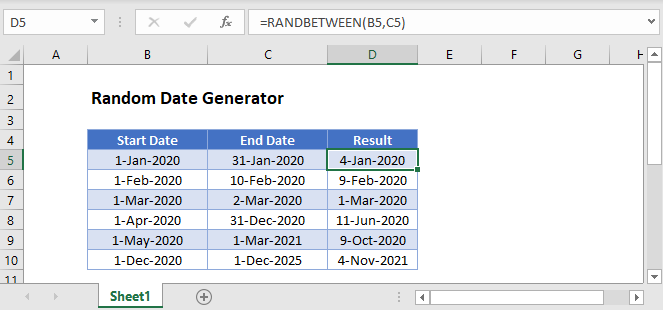## Random Date Generator

For various simulations, you may need to generate a random date. To do this in Excel, use the RANDBETWEEN Function or, If you’re working in Excel for Microsoft 365 (or versions of Excel after 2019), you have the option to use the RANDARRAY Function instead.

### RANDBETWEEN Function

The RANDBETWEEN Function generates a random number between two defined values.

In this example, we use dates for the RANDBETWEEN limits to return a random date in the year 2020.

``=RANDBETWEEN(DATE(2020,12,1),DATE(2020,12,31))``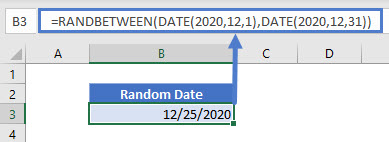You can use the DATE function to generate your limit dates, or you can refer to date cells instead:

``=RANDBETWEEN(B3,C3)``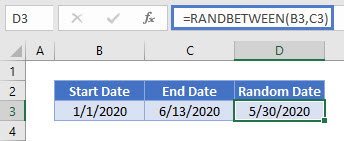## Generate Random Workdays

You can also generate random workdays by combining the WORKDAY and RANDBETWEEN functions.

``=WORKDAY(RANDBETWEEN(B3,C3)-1,1)``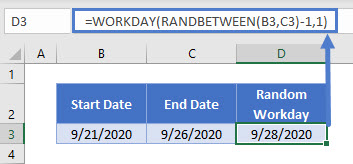Using the WORKDAY function with the arguments above shifts dates that fall on weekends (or holidays) to the next working day, so the generated random workday can be one or two days later than the end date.

## RANDARRAY Function

The RANDARRAY Function is available only in Excel for Microsoft 365 (versions of Excel after 2019). It can generate an array of random numbers with the specified number of rows and columns, minimum and maximum values, and with decimal values (FALSE) or whole numbers only (TRUE). Its syntax is: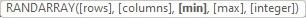``=RANDARRAY(2,3,B3,C3,TRUE)``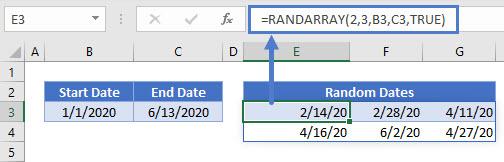This example generates a 2-row, 3-column array filled with dates (if cells are formatted accordingly) between the dates specified in the referenced cells. You only need to enter the formula in the first cell and don’t need to use CTRL + SHIFT + ENTER as you would normally have to for arrays.

Please note that all the above random numbers will be regenerated each time the workbook is opened or changed, unless the calculation options are set to manual.

## Google Sheets –Random Date Generator

All of the above examples work exactly the same in Google Sheets as in Excel.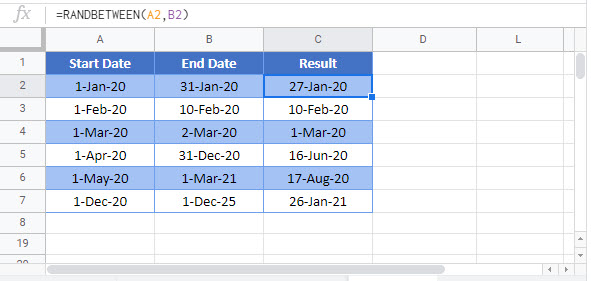### Excel Practice Worksheet

Practice Excel functions and formulas with our 100% free practice worksheets!